Középiskolai Matematikai és Fizikai Lapok
Informatika rovattal
 Már regisztráltál? Új vendég vagy?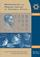# Angol nyelvű szám, 2002. december

Előző oldalTartalomjegyzékKövetkező oldalMEGRENDELŐLAP

## Solutions of problems B

B. 3471. An ant is walking about in the region bounded by the curve of equation x2+y2+xy=6. Its path consists of segments parallel to the coordinate axes. Starting at an arbitrary point of the curve it walks straight ahead inside the curve until it hits the boundary again. Then it makes a 90o turn and goes on walking inside the curve. It only stops if it either arrives at a point of the boundary where it has been before, or if it cannot continue its walk by the above rules. Prove that wherever the starting point is, the walk of the ant will always terminate.

Gillis-Turán Mathematics Competition, 2000

Solution 1. If the ant is at a point P(a,b) of the curve, then the equations x2+bx+b2-6=0 and y2+ay+a2-6=0 have real roots: a and b, respectively. Thus the discriminants Db= 24-3b2 and Da=24-3a2 are non-negative. If either of them is zero, for example Da=0, that is $\displaystyle |a|=2\sqrt{2}$ (hence $\displaystyle b=-\frac{a}{2}$) then the ant cannot continue its walk parallel to the y-axis. This happens at the points $\displaystyle Y_1\big(2\sqrt{2},-\sqrt{2}\,\big)$ and $\displaystyle Y_2\big(-2\sqrt{2},\sqrt{2}\,\big)$. Similarly, when Db=0, that is, the ant is at the points $\displaystyle X_1\big(\sqrt{2}, -\sqrt{2}\,\big)$ or $\displaystyle X_2\big(-\sqrt{2},2\sqrt{2}\,\big)$, it cannot continue its walk parallel to the x-axis.

This is all clearly seen if one considers that the curve x2+y2+xy=6 is an ellipse (Figure 1), and the points Xi, Yi are the points where the tangents parallel to the coordinate axes touch the curve.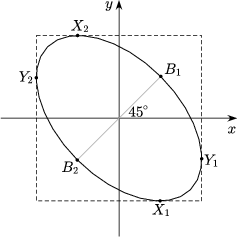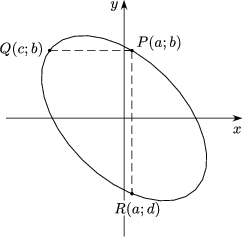Figure 1 Figure 2

If the ant is at a point P(a,b) different from these four points then it can walk in either allowed direction. If P is not the starting point, then it can obviously walk in one direction only, as it must have come from the other direction.

For example, if the ant is walking parallel to the x-axis, and it arrives at a point Q(c,b) of the curve then with the above reasoning c$\displaystyle \ne$a, and the two numbers a and c are the two real roots of the equation x2+bx+b2-6=0. Thus a+c=-b.

Similarly, if the ant is walking parallel to the y-axis and arrives at a point R(a,d) from P then d+b=-a (Figure 2).

It follows that the endpoints of any segment of the ant's walk are such points of the curve that two out of the 4 coordinates altogether are equal, and the sum of this coordinate and the remaining two is 0: a+b+c=0 and a+b+d=0.

For example, if the ant starts out at P0(a,b) parallel to the x-axis, its path will pass through the following points of the curve:

A. Babos, Budapest

Remarks. 1. It is easy to see that the ant can only arrive at the point Xi if it is walking parallel to the y-axis: to X1 from $\displaystyle B_1\big(\sqrt{2},\sqrt{2}\,\big)$ and to X2 from $\displaystyle B_2\big(-\sqrt{2},-\sqrt{2}\,\big)$. These are the same points, with their coordinates equal, from where the ant can reach Y1 and Y2.

To summarize the results, we can state that if the starting position of the ant is different from the points Xi, Yi, Bi then its walk will terminate in six steps, if it starts from Bi then it will terminate in one step, and if it starts from Xi or Yi then two steps. All principally correct solutions used more or less the same reasoning, but it eluded almost everyone to examine the special cases.

2. With the notation c=-a-b, the path of the ant (with the exception of the special cases) touches the boundary at the points (a,b), (c,b), (c,a), (b,a), (b,c), (a,c), (a,b). The coordinates of the points are all a, b or c where a+b+c=0 (Figure 3). The path, just like the curve itself, is symmetrical in the line y=x.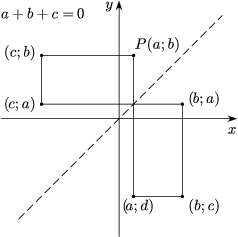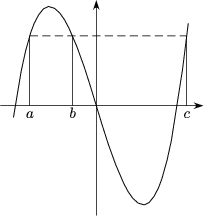Figure 3 Figure 4

The number triples of this kind are in an interesting relationship to a possible approach of problem B. 3470. (See the Remark to Problem B. 3470 on page 51.) If the original polynomial is the third-degree polynomial f(x)= x3-6x (Figure 4) then the equation of the ellipse of this problem is $\displaystyle \frac{f(x)-f(y)}{x-y}=0$, and the coordinates of the points of the ellipse are number pairs (a,b) for which f(a)=f(b).

Each value of f other than the local extrema is assumed at three points, and the path of the ant corresponds to a number triple of this kind. With this interpretation, the statement of the problem is obvious.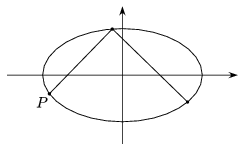Figure 5

Solution 2. Let us rotate the curve, as well as the path of the ant, through -45o. Then the equation of the curve will be

$\displaystyle \frac{x^2}{12}+\frac{y^2}{4}=1$

(Figure 5).

The rotated path consist of segments enclosing 45o with the coordinate axes. The eccentricity of the ellipse is $\displaystyle \frac{b}{a}=\frac{1}{\sqrt{3}}$, therefore an orthogonal axial affinity with a scale factor of $\displaystyle \sqrt{3}$ applied to the ellipse and the ant's path will map the ellipse onto a circle centred at the origin, and the path of the ant onto a polygon of segments enclosing +60o and -60o with the x-axis alternately (Figure 6).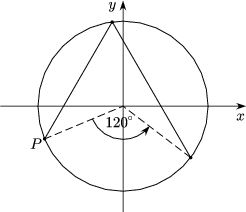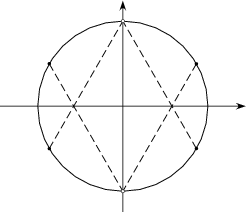Figure 6 Figure 7

Thus the position of the ant on the curve after two steps can be obtained by rotating the starting point through 120o about the origin. The direction of the rotation depends on the direction of the ant's walk, and since the direction stays the same all through the ant's journey, the ant will necessarily return to its starting position in six consecutive steps, and that terminates its walk.

This is true for almost all starting points, with the exception of six points. Four of these are the points where the tangents enclose angles of 60o or -60o with the x-axis. If the ant arrives at these points, it must stop. The other two are the endpoints of the vertical diameter, the points from where the ant can reach those points of tangency (Figure 7).